﻿ 基于有限差分法的承压稳定井流计算方法的比较 Comparison of Calculation Methods for Confined Steady Well Flow Based on Finite Difference Method

Advances in Applied Mathematics
Vol.06 No.09(2017), Article ID:23177,6 pages
10.12677/AAM.2017.69145

Comparison of Calculation Methods for Confined Steady Well Flow Based on Finite Difference Method

Siyu Zhang, Deliang Zhou, Jingyi Sun

School of Mathematics, Liaoning Normal University, Dalian Liaoning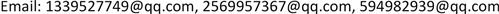Received: Dec. 3rd, 2017; accepted: Dec. 20th, 2017; published: Dec. 27th, 2017ABSTRACT

Based on finite difference method, we use early method, well level optimization method and polishing singular point method to calculate the well level in example respectively. The example is calculated and compared. The well level calculated by polishing singular point method is closest to the real well level.

Keywords:Finite Difference Method, Well Level Calculation, Comparison, Polishing Singular Point MethodCopyright © 2017 by authors and Hans Publishers Inc.1. 引言

2. 井水位早期计算方法

$\left\{\begin{array}{l}-T\Delta u=f\left(x,y\right),\text{\hspace{0.17em}}\text{\hspace{0.17em}}x,y\in G\text{\hspace{0.17em}}\text{\hspace{0.17em}}\text{\hspace{0.17em}}\text{\hspace{0.17em}}\text{\hspace{0.17em}}\text{\hspace{0.17em}}\text{\hspace{0.17em}}\text{\hspace{0.17em}}\text{\hspace{0.17em}}\text{\hspace{0.17em}}\text{\hspace{0.17em}}\text{\hspace{0.17em}}\text{\hspace{0.17em}}\text{\hspace{0.17em}}\text{\hspace{0.17em}}\text{\hspace{0.17em}}\text{\hspace{0.17em}}\text{\hspace{0.17em}}\text{\hspace{0.17em}}\text{\hspace{0.17em}}\text{\hspace{0.17em}}\text{\hspace{0.17em}}\text{\hspace{0.17em}}\text{\hspace{0.17em}}\text{\hspace{0.17em}}\text{\hspace{0.17em}}\text{\hspace{0.17em}}\text{\hspace{0.17em}}\text{\hspace{0.17em}}\text{\hspace{0.17em}}\text{\hspace{0.17em}}\text{\hspace{0.17em}}\text{\hspace{0.17em}}\text{\hspace{0.17em}}\text{\hspace{0.17em}}\text{\hspace{0.17em}}\text{\hspace{0.17em}}\text{\hspace{0.17em}}\text{\hspace{0.17em}}\text{\hspace{0.17em}}\text{\hspace{0.17em}}\text{\hspace{0.17em}}\text{\hspace{0.17em}}\text{\hspace{0.17em}}\text{\hspace{0.17em}}\text{ }\text{\hspace{0.17em}}\text{\hspace{0.17em}}\text{}\left(2.1\right)\\ {u|}_{{\Gamma }_{1}}=a\left(x,y\right),\text{\hspace{0.17em}}\text{\hspace{0.17em}}在边界{\Gamma }_{1}上\text{\hspace{0.17em}}\text{\hspace{0.17em}}\text{\hspace{0.17em}}\text{\hspace{0.17em}}\text{\hspace{0.17em}}\text{\hspace{0.17em}}\text{\hspace{0.17em}}\text{\hspace{0.17em}}\text{\hspace{0.17em}}\text{\hspace{0.17em}}\text{\hspace{0.17em}}\text{\hspace{0.17em}}\text{\hspace{0.17em}}\text{\hspace{0.17em}}\text{\hspace{0.17em}}\text{\hspace{0.17em}}\text{\hspace{0.17em}}\text{\hspace{0.17em}}\text{\hspace{0.17em}}\text{\hspace{0.17em}}\text{\hspace{0.17em}}\text{\hspace{0.17em}}\text{\hspace{0.17em}}\text{\hspace{0.17em}}\text{\hspace{0.17em}}\text{\hspace{0.17em}}\text{\hspace{0.17em}}\text{\hspace{0.17em}}\text{\hspace{0.17em}}\text{\hspace{0.17em}}\text{\hspace{0.17em}}\text{\hspace{0.17em}}\text{\hspace{0.17em}}\text{\hspace{0.17em}}\text{\hspace{0.17em}}\text{\hspace{0.17em}}\text{\hspace{0.17em}}\text{\hspace{0.17em}}\text{\hspace{0.17em}}\text{ }\text{\hspace{0.17em}}\text{\hspace{0.17em}}\text{\hspace{0.17em}}\text{\hspace{0.17em}}\text{\hspace{0.17em}}\text{\hspace{0.17em}}\text{}\left(2.2\right)\\ {T\frac{\partial u}{\partial n}|}_{{\Gamma }_{2}}=b\left(x,y\right),\text{\hspace{0.17em}}\text{\hspace{0.17em}}在边界{\Gamma }_{2}上\text{\hspace{0.17em}}\text{\hspace{0.17em}}\text{\hspace{0.17em}}\text{\hspace{0.17em}}\text{\hspace{0.17em}}\text{\hspace{0.17em}}\text{\hspace{0.17em}}\text{\hspace{0.17em}}\text{\hspace{0.17em}}\text{\hspace{0.17em}}\text{\hspace{0.17em}}\text{\hspace{0.17em}}\text{\hspace{0.17em}}\text{\hspace{0.17em}}\text{\hspace{0.17em}}\text{\hspace{0.17em}}\text{\hspace{0.17em}}\text{\hspace{0.17em}}\text{\hspace{0.17em}}\text{\hspace{0.17em}}\text{\hspace{0.17em}}\text{\hspace{0.17em}}\text{\hspace{0.17em}}\text{\hspace{0.17em}}\text{\hspace{0.17em}}\text{\hspace{0.17em}}\text{\hspace{0.17em}}\text{\hspace{0.17em}}\text{\hspace{0.17em}}\text{\hspace{0.17em}}\text{\hspace{0.17em}}\text{\hspace{0.17em}}\text{\hspace{0.17em}}\text{\hspace{0.17em}}\text{\hspace{0.17em}}\text{\hspace{0.17em}}\text{\hspace{0.17em}}\text{\hspace{0.17em}}\text{\hspace{0.17em}}\text{\hspace{0.17em}}\text{}\left(2.3\right)\\ {T\frac{\partial u}{\partial n}|}_{{C}_{k}}=-\frac{{q}_{k}}{2\text{π}{\rho }_{k}},\text{\hspace{0.17em}}\text{\hspace{0.17em}}在井边界{C}_{k}上,\text{\hspace{0.17em}}\text{\hspace{0.17em}}k=1,2,\cdots ,n\text{\hspace{0.17em}}\text{\hspace{0.17em}}\text{\hspace{0.17em}}\text{\hspace{0.17em}}\text{\hspace{0.17em}}\text{\hspace{0.17em}}\text{\hspace{0.17em}}\text{\hspace{0.17em}}\text{\hspace{0.17em}}\text{\hspace{0.17em}}\text{\hspace{0.17em}}\text{\hspace{0.17em}}\text{\hspace{0.17em}}\text{\hspace{0.17em}}\text{\hspace{0.17em}}\text{\hspace{0.17em}}\text{ }\text{}\left(2.4\right)\end{array}$

$\begin{array}{c}-\Delta u=-\left(\frac{{u}_{i+1,j}-2{u}_{i,j}+{u}_{i-1,j}}{{h}_{x}{}^{2}}+\frac{{u}_{i,j+1}-2{u}_{i,j}+{u}_{i,j-1}}{{h}_{y}{}^{2}}\right)\\ =-\frac{1}{{h}_{x}^{2}}{u}_{i+1,j}-\frac{1}{{h}_{x}^{2}}{u}_{i-1,j}-\frac{1}{{h}_{y}^{2}}{u}_{i,j+1}-\frac{1}{{h}_{y}^{2}}{u}_{i,j-1}+2\left(\frac{1}{{h}_{x}^{2}}+\frac{1}{{h}_{y}^{2}}\right){u}_{i,j}\end{array}$

$\left\{\begin{array}{l}T\left[-\frac{1}{{h}_{x}^{2}}{u}_{i+1,j}-\frac{1}{{h}_{x}^{2}}{u}_{i-1,j}-\frac{1}{{h}_{y}^{2}}{u}_{i,j+1}-\frac{1}{{h}_{y}^{2}}{u}_{i,j-1}+2\left(\frac{1}{{h}_{x}^{2}}+\frac{1}{{h}_{y}^{2}}\right){u}_{i,j}\right]=f,\text{\hspace{0.17em}}\text{\hspace{0.17em}}在区域G上\text{\hspace{0.17em}}\text{\hspace{0.17em}}\text{\hspace{0.17em}}\text{\hspace{0.17em}}\text{\hspace{0.17em}}\text{\hspace{0.17em}}\text{\hspace{0.17em}}\text{\hspace{0.17em}}\text{\hspace{0.17em}}\text{\hspace{0.17em}}\text{\hspace{0.17em}}\left(2.5\right)\\ {u|}_{{\Gamma }_{1}}=a\left(x,y\right),\text{\hspace{0.17em}}\text{\hspace{0.17em}}在边界{\Gamma }_{1}上\text{\hspace{0.17em}}\text{\hspace{0.17em}}\text{\hspace{0.17em}}\text{\hspace{0.17em}}\text{\hspace{0.17em}}\text{\hspace{0.17em}}\text{\hspace{0.17em}}\text{\hspace{0.17em}}\text{\hspace{0.17em}}\text{\hspace{0.17em}}\text{\hspace{0.17em}}\text{\hspace{0.17em}}\text{\hspace{0.17em}}\text{\hspace{0.17em}}\text{\hspace{0.17em}}\text{\hspace{0.17em}}\text{\hspace{0.17em}}\text{\hspace{0.17em}}\text{\hspace{0.17em}}\text{\hspace{0.17em}}\text{\hspace{0.17em}}\text{\hspace{0.17em}}\text{\hspace{0.17em}}\text{\hspace{0.17em}}\text{\hspace{0.17em}}\text{\hspace{0.17em}}\text{\hspace{0.17em}}\text{\hspace{0.17em}}\text{\hspace{0.17em}}\text{\hspace{0.17em}}\text{\hspace{0.17em}}\text{\hspace{0.17em}}\text{\hspace{0.17em}}\text{\hspace{0.17em}}\text{\hspace{0.17em}}\text{\hspace{0.17em}}\text{\hspace{0.17em}}\text{\hspace{0.17em}}\text{\hspace{0.17em}}\text{\hspace{0.17em}}\text{\hspace{0.17em}}\text{\hspace{0.17em}}\text{\hspace{0.17em}}\text{\hspace{0.17em}}\text{\hspace{0.17em}}\text{\hspace{0.17em}}\text{\hspace{0.17em}}\text{\hspace{0.17em}}\text{\hspace{0.17em}}\text{\hspace{0.17em}}\text{\hspace{0.17em}}\text{\hspace{0.17em}}\text{\hspace{0.17em}}\text{\hspace{0.17em}}\text{\hspace{0.17em}}\text{\hspace{0.17em}}\text{\hspace{0.17em}}\text{\hspace{0.17em}}\text{\hspace{0.17em}}\text{\hspace{0.17em}}\text{\hspace{0.17em}}\text{\hspace{0.17em}}\text{\hspace{0.17em}}\text{\hspace{0.17em}}\text{\hspace{0.17em}}\text{\hspace{0.17em}}\text{\hspace{0.17em}}\text{\hspace{0.17em}}\text{\hspace{0.17em}}\text{\hspace{0.17em}}\text{\hspace{0.17em}}\text{\hspace{0.17em}}\text{\hspace{0.17em}}\text{\hspace{0.17em}}\text{\hspace{0.17em}}\text{\hspace{0.17em}}\text{\hspace{0.17em}}\text{\hspace{0.17em}}\text{\hspace{0.17em}}\text{\hspace{0.17em}}\text{\hspace{0.17em}}\text{\hspace{0.17em}}\text{\hspace{0.17em}}\left(2.6\right)\\ {T\left(\frac{{u}_{i,j+1}-2{u}_{i,j}+{u}_{i,j-1}}{{h}_{y}^{2}}\right)|}_{{\Gamma }_{2}}=B\left(x,y\right),\text{\hspace{0.17em}}\text{\hspace{0.17em}}在边界{\Gamma }_{2}和{C}_{k}上\text{\hspace{0.17em}}\text{\hspace{0.17em}}\text{\hspace{0.17em}}\text{\hspace{0.17em}}\text{\hspace{0.17em}}\text{\hspace{0.17em}}\text{\hspace{0.17em}}\text{\hspace{0.17em}}\text{\hspace{0.17em}}\text{\hspace{0.17em}}\text{\hspace{0.17em}}\text{\hspace{0.17em}}\text{\hspace{0.17em}}\text{\hspace{0.17em}}\text{\hspace{0.17em}}\text{\hspace{0.17em}}\text{\hspace{0.17em}}\text{\hspace{0.17em}}\text{\hspace{0.17em}}\text{\hspace{0.17em}}\text{\hspace{0.17em}}\text{\hspace{0.17em}}\text{\hspace{0.17em}}\text{\hspace{0.17em}}\text{\hspace{0.17em}}\text{\hspace{0.17em}}\text{\hspace{0.17em}}\text{\hspace{0.17em}}\text{\hspace{0.17em}}\text{\hspace{0.17em}}\text{\hspace{0.17em}}\text{\hspace{0.17em}}\text{\hspace{0.17em}}\text{\hspace{0.17em}}\text{\hspace{0.17em}}\text{\hspace{0.17em}}\text{\hspace{0.17em}}\text{\hspace{0.17em}}\text{\hspace{0.17em}}\text{\hspace{0.17em}}\text{\hspace{0.17em}}\text{\hspace{0.17em}}\text{\hspace{0.17em}}\text{\hspace{0.17em}}\left(2.7\right)\end{array}$

$\left\{\begin{array}{l}\frac{\partial }{\partial x}\left(T\frac{\partial u}{\partial x}\right)+\frac{\partial }{\partial y}\left(T\frac{\partial u}{\partial y}\right)=-\sum _{k=1}^{n}{q}_{k}\delta \left(x-{\overline{x}}_{k},y-{\overline{y}}_{k}\right)+\epsilon \left(x,y\right),\text{\hspace{0.17em}}\text{\hspace{0.17em}}在区域G上\text{\hspace{0.17em}}\text{\hspace{0.17em}}\text{\hspace{0.17em}}\text{\hspace{0.17em}}\text{\hspace{0.17em}}\text{\hspace{0.17em}}\text{\hspace{0.17em}}\text{\hspace{0.17em}}\text{\hspace{0.17em}}\text{\hspace{0.17em}}\text{\hspace{0.17em}}\text{\hspace{0.17em}}\text{\hspace{0.17em}}\text{\hspace{0.17em}}\text{\hspace{0.17em}}\text{\hspace{0.17em}}\text{\hspace{0.17em}}\text{ }\left(2.8\right)\\ u|{}_{{\Gamma }_{1}}=a\left(x,y\right),\text{\hspace{0.17em}}\text{\hspace{0.17em}}在边界{\Gamma }_{1}上\text{\hspace{0.17em}}\text{\hspace{0.17em}}\text{\hspace{0.17em}}\text{\hspace{0.17em}}\text{\hspace{0.17em}}\text{\hspace{0.17em}}\text{\hspace{0.17em}}\text{\hspace{0.17em}}\text{\hspace{0.17em}}\text{\hspace{0.17em}}\text{\hspace{0.17em}}\text{\hspace{0.17em}}\text{\hspace{0.17em}}\text{\hspace{0.17em}}\text{\hspace{0.17em}}\text{\hspace{0.17em}}\text{\hspace{0.17em}}\text{\hspace{0.17em}}\text{\hspace{0.17em}}\text{\hspace{0.17em}}\text{\hspace{0.17em}}\text{\hspace{0.17em}}\text{\hspace{0.17em}}\text{\hspace{0.17em}}\text{\hspace{0.17em}}\text{\hspace{0.17em}}\text{\hspace{0.17em}}\text{\hspace{0.17em}}\text{\hspace{0.17em}}\text{\hspace{0.17em}}\text{\hspace{0.17em}}\text{\hspace{0.17em}}\text{\hspace{0.17em}}\text{\hspace{0.17em}}\text{\hspace{0.17em}}\text{\hspace{0.17em}}\text{\hspace{0.17em}}\text{\hspace{0.17em}}\text{\hspace{0.17em}}\text{\hspace{0.17em}}\text{\hspace{0.17em}}\text{\hspace{0.17em}}\text{\hspace{0.17em}}\text{\hspace{0.17em}}\text{\hspace{0.17em}}\text{\hspace{0.17em}}\text{\hspace{0.17em}}\text{\hspace{0.17em}}\text{\hspace{0.17em}}\text{\hspace{0.17em}}\text{\hspace{0.17em}}\text{\hspace{0.17em}}\text{\hspace{0.17em}}\text{\hspace{0.17em}}\text{\hspace{0.17em}}\text{\hspace{0.17em}}\text{\hspace{0.17em}}\text{\hspace{0.17em}}\text{\hspace{0.17em}}\text{\hspace{0.17em}}\text{\hspace{0.17em}}\text{\hspace{0.17em}}\text{\hspace{0.17em}}\text{\hspace{0.17em}}\text{\hspace{0.17em}}\text{\hspace{0.17em}}\text{\hspace{0.17em}}\text{\hspace{0.17em}}\text{\hspace{0.17em}}\text{\hspace{0.17em}}\text{\hspace{0.17em}}\text{ }\text{\hspace{0.17em}}\text{\hspace{0.17em}}\text{\hspace{0.17em}}\text{\hspace{0.17em}}\text{\hspace{0.17em}}\text{\hspace{0.17em}}\text{\hspace{0.17em}}\text{\hspace{0.17em}}\left(2.9\right)\\ {T\frac{\partial u}{\partial n}|}_{{\Gamma }_{2}}=b\left(x,y\right),\text{\hspace{0.17em}}\text{\hspace{0.17em}}在边界{\Gamma }_{2}上\text{\hspace{0.17em}}\text{\hspace{0.17em}}\text{\hspace{0.17em}}\text{\hspace{0.17em}}\text{\hspace{0.17em}}\text{\hspace{0.17em}}\text{\hspace{0.17em}}\text{\hspace{0.17em}}\text{\hspace{0.17em}}\text{\hspace{0.17em}}\text{\hspace{0.17em}}\text{\hspace{0.17em}}\text{\hspace{0.17em}}\text{\hspace{0.17em}}\text{\hspace{0.17em}}\text{\hspace{0.17em}}\text{\hspace{0.17em}}\text{\hspace{0.17em}}\text{\hspace{0.17em}}\text{\hspace{0.17em}}\text{\hspace{0.17em}}\text{\hspace{0.17em}}\text{\hspace{0.17em}}\text{\hspace{0.17em}}\text{\hspace{0.17em}}\text{\hspace{0.17em}}\text{\hspace{0.17em}}\text{\hspace{0.17em}}\text{\hspace{0.17em}}\text{\hspace{0.17em}}\text{\hspace{0.17em}}\text{\hspace{0.17em}}\text{\hspace{0.17em}}\text{\hspace{0.17em}}\text{\hspace{0.17em}}\text{\hspace{0.17em}}\text{\hspace{0.17em}}\text{\hspace{0.17em}}\text{\hspace{0.17em}}\text{\hspace{0.17em}}\text{\hspace{0.17em}}\text{\hspace{0.17em}}\text{\hspace{0.17em}}\text{\hspace{0.17em}}\text{\hspace{0.17em}}\text{\hspace{0.17em}}\text{\hspace{0.17em}}\text{\hspace{0.17em}}\text{\hspace{0.17em}}\text{\hspace{0.17em}}\text{\hspace{0.17em}}\text{\hspace{0.17em}}\text{\hspace{0.17em}}\text{\hspace{0.17em}}\text{\hspace{0.17em}}\text{\hspace{0.17em}}\text{\hspace{0.17em}}\text{\hspace{0.17em}}\text{\hspace{0.17em}}\text{\hspace{0.17em}}\text{\hspace{0.17em}}\text{\hspace{0.17em}}\text{\hspace{0.17em}}\text{\hspace{0.17em}}\text{\hspace{0.17em}}\text{\hspace{0.17em}}\text{\hspace{0.17em}}\text{\hspace{0.17em}}\text{\hspace{0.17em}}\text{\hspace{0.17em}}\text{\hspace{0.17em}}\text{\hspace{0.17em}}\text{\hspace{0.17em}}\left(2.10\right)\end{array}$

3. 井水位优化方法

${u}_{j}={u}_{w}+\frac{Q}{2\text{π}T}\mathrm{ln}\frac{\Delta x}{{r}_{w}}$ (3.1)

${u}_{m}={u}_{w}+\frac{Q}{2\text{π}T}\mathrm{ln}\frac{\Delta y}{{r}_{w}}$ (3.2)

${u}_{k}={u}_{w}+\frac{Q}{2\text{π}T}\mathrm{ln}\frac{\Delta x}{{r}_{w}}$ (3.3)

${u}_{n}={u}_{w}+\frac{Q}{2\text{π}T}\mathrm{ln}\frac{\Delta y}{{r}_{w}}$ (3.4)

$Q=T\Delta x\left[\frac{{u}_{m}-{u}_{i}}{\Delta y}+\frac{{u}_{n}-{u}_{i}}{\Delta y}\right]+T\Delta y\left[\frac{{u}_{j}-{u}_{i}}{\Delta x}+\frac{{u}_{k}-{u}_{i}}{\Delta x}\right]$ (3.5)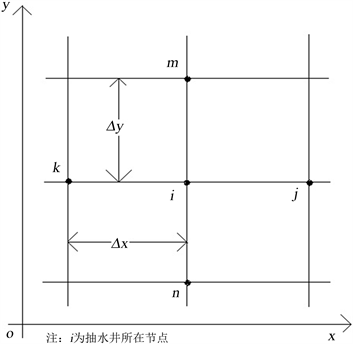Figure 1. Plane difference grid near well point

${u}_{w}={u}_{i}-\frac{Q}{2\text{π}T}\frac{\Delta x\Delta y}{\Delta {x}^{2}+\Delta {y}^{2}}\left[\frac{\Delta y}{\Delta x}\mathrm{ln}\frac{\Delta x}{{r}_{w}}+\frac{\Delta x}{\Delta y}\mathrm{ln}\frac{\Delta y}{{r}_{w}}-\text{π}\right]$ (3.6)

${u}_{w}={u}_{i}-\frac{Q}{2\text{π}T}\left(\mathrm{ln}\frac{\Delta x}{{r}_{w}}-\frac{\text{π}}{2}\right)$ (3.7)

4. 奇点磨光法

$w=u-v=u-\sum _{k=1}^{n}{v}_{k}$ ，对于问题(2.1)~(2.4)就转变为求解下述问题

$\left\{\begin{array}{l}\frac{\partial }{\partial x}\left(T\frac{\partial w}{\partial x}\right)+\frac{\partial }{\partial y}\left(T\frac{\partial w}{\partial y}\right)=-f-\left(\frac{\partial }{\partial x}\left(T\frac{\partial v}{\partial x}\right)+\frac{\partial }{\partial y}\left(T\frac{\partial v}{\partial y}\right)\right),\text{\hspace{0.17em}}\text{\hspace{0.17em}}在区域\Omega 上\text{\hspace{0.17em}}\text{\hspace{0.17em}}\text{\hspace{0.17em}}\text{\hspace{0.17em}}\text{\hspace{0.17em}}\text{\hspace{0.17em}}\text{\hspace{0.17em}}\text{\hspace{0.17em}}\text{\hspace{0.17em}}\text{\hspace{0.17em}}\text{\hspace{0.17em}}\text{\hspace{0.17em}}\text{\hspace{0.17em}}\text{\hspace{0.17em}}\text{\hspace{0.17em}}\text{\hspace{0.17em}}\text{\hspace{0.17em}}\text{\hspace{0.17em}}\text{\hspace{0.17em}}\text{\hspace{0.17em}}\text{\hspace{0.17em}}\text{\hspace{0.17em}}\text{\hspace{0.17em}}\text{\hspace{0.17em}}\text{ }\left(4.1\right)\\ {w\left(x,y\right)|}_{{\Gamma }_{1}}=a\left(x,y\right),\text{\hspace{0.17em}}\text{\hspace{0.17em}}在边界{\Gamma }_{1}上\text{\hspace{0.17em}}\text{\hspace{0.17em}}\text{\hspace{0.17em}}\text{\hspace{0.17em}}\text{\hspace{0.17em}}\text{\hspace{0.17em}}\text{\hspace{0.17em}}\text{\hspace{0.17em}}\text{\hspace{0.17em}}\text{\hspace{0.17em}}\text{\hspace{0.17em}}\text{\hspace{0.17em}}\text{\hspace{0.17em}}\text{\hspace{0.17em}}\text{\hspace{0.17em}}\text{\hspace{0.17em}}\text{\hspace{0.17em}}\text{\hspace{0.17em}}\text{\hspace{0.17em}}\text{\hspace{0.17em}}\text{\hspace{0.17em}}\text{\hspace{0.17em}}\text{\hspace{0.17em}}\text{\hspace{0.17em}}\text{\hspace{0.17em}}\text{\hspace{0.17em}}\text{\hspace{0.17em}}\text{\hspace{0.17em}}\text{\hspace{0.17em}}\text{\hspace{0.17em}}\text{\hspace{0.17em}}\text{\hspace{0.17em}}\text{\hspace{0.17em}}\text{\hspace{0.17em}}\text{\hspace{0.17em}}\text{\hspace{0.17em}}\text{\hspace{0.17em}}\text{\hspace{0.17em}}\text{\hspace{0.17em}}\text{\hspace{0.17em}}\text{\hspace{0.17em}}\text{\hspace{0.17em}}\text{\hspace{0.17em}}\text{\hspace{0.17em}}\text{\hspace{0.17em}}\text{\hspace{0.17em}}\text{\hspace{0.17em}}\text{\hspace{0.17em}}\text{\hspace{0.17em}}\text{\hspace{0.17em}}\text{\hspace{0.17em}}\text{\hspace{0.17em}}\text{\hspace{0.17em}}\text{\hspace{0.17em}}\text{\hspace{0.17em}}\text{\hspace{0.17em}}\text{\hspace{0.17em}}\text{\hspace{0.17em}}\text{\hspace{0.17em}}\text{\hspace{0.17em}}\text{\hspace{0.17em}}\text{\hspace{0.17em}}\text{\hspace{0.17em}}\text{\hspace{0.17em}}\text{\hspace{0.17em}}\text{\hspace{0.17em}}\text{\hspace{0.17em}}\text{\hspace{0.17em}}\text{\hspace{0.17em}}\text{\hspace{0.17em}}\text{\hspace{0.17em}}\text{\hspace{0.17em}}\text{\hspace{0.17em}}\text{\hspace{0.17em}}\text{\hspace{0.17em}}\text{\hspace{0.17em}}\text{ }\left(4.2\right)\\ {T\frac{\partial w}{\partial n}|}_{{\Gamma }_{2}}=b\left(x,y\right),\text{\hspace{0.17em}}\text{\hspace{0.17em}}在边界{\Gamma }_{2}上\text{\hspace{0.17em}}\text{\hspace{0.17em}}\text{\hspace{0.17em}}\text{\hspace{0.17em}}\text{\hspace{0.17em}}\text{\hspace{0.17em}}\text{\hspace{0.17em}}\text{\hspace{0.17em}}\text{\hspace{0.17em}}\text{\hspace{0.17em}}\text{\hspace{0.17em}}\text{\hspace{0.17em}}\text{\hspace{0.17em}}\text{\hspace{0.17em}}\text{\hspace{0.17em}}\text{\hspace{0.17em}}\text{\hspace{0.17em}}\text{\hspace{0.17em}}\text{\hspace{0.17em}}\text{\hspace{0.17em}}\text{\hspace{0.17em}}\text{\hspace{0.17em}}\text{\hspace{0.17em}}\text{\hspace{0.17em}}\text{\hspace{0.17em}}\text{\hspace{0.17em}}\text{\hspace{0.17em}}\text{\hspace{0.17em}}\text{\hspace{0.17em}}\text{\hspace{0.17em}}\text{\hspace{0.17em}}\text{\hspace{0.17em}}\text{\hspace{0.17em}}\text{\hspace{0.17em}}\text{\hspace{0.17em}}\text{\hspace{0.17em}}\text{\hspace{0.17em}}\text{\hspace{0.17em}}\text{\hspace{0.17em}}\text{\hspace{0.17em}}\text{\hspace{0.17em}}\text{\hspace{0.17em}}\text{\hspace{0.17em}}\text{\hspace{0.17em}}\text{\hspace{0.17em}}\text{\hspace{0.17em}}\text{\hspace{0.17em}}\text{\hspace{0.17em}}\text{\hspace{0.17em}}\text{\hspace{0.17em}}\text{\hspace{0.17em}}\text{\hspace{0.17em}}\text{\hspace{0.17em}}\text{\hspace{0.17em}}\text{\hspace{0.17em}}\text{\hspace{0.17em}}\text{\hspace{0.17em}}\text{\hspace{0.17em}}\text{\hspace{0.17em}}\text{\hspace{0.17em}}\text{\hspace{0.17em}}\text{\hspace{0.17em}}\text{\hspace{0.17em}}\text{\hspace{0.17em}}\text{\hspace{0.17em}}\text{\hspace{0.17em}}\text{\hspace{0.17em}}\text{\hspace{0.17em}}\text{\hspace{0.17em}}\text{\hspace{0.17em}}\text{\hspace{0.17em}}\text{\hspace{0.17em}}\text{\hspace{0.17em}}\text{\hspace{0.17em}}\text{\hspace{0.17em}}\text{\hspace{0.17em}}\text{\hspace{0.17em}}\text{\hspace{0.17em}}\text{\hspace{0.17em}}\text{ }\left(4.3\right)\end{array}$

$f$ 光滑无奇性。为保证求解精度，函数 ${v}_{k}$ 的选择除了要满足前述4个条件外，还要能使方程(4.1)的右端项 $\frac{\partial }{\partial x}\left(T\frac{\partial v}{\partial x}\right)+\frac{\partial }{\partial y}\left(T\frac{\partial v}{\partial y}\right)$ 无奇性且连续。

5. 数值算例

$v=\mathrm{ln}r-\left(r-1\right)+\frac{1}{2}{\left(r-1\right)}^{2}+\frac{2}{3}{\left(r-1\right)}^{3}\text{\hspace{0.17em}}\left(r$v=0\text{\hspace{0.17em}}\left(r\ge R\right)$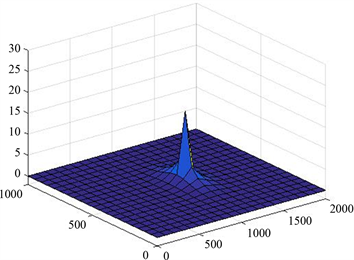Figure 2. Early method approximate water level and true water level error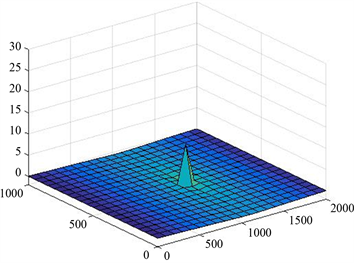Figure 3. Water level optimization method approximate water level and true water level error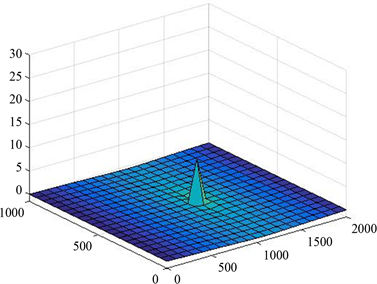Figure 4. Approximate water level by singular point smoothing method and true water level error

6. 结语

Comparison of Calculation Methods for Confined Steady Well Flow Based on Finite Difference Method[J]. 应用数学进展, 2017, 06(09): 1201-1206. http://dx.doi.org/10.12677/AAM.2017.69145

1. 1. 张宏仁. 解地下水渗流问题的有限差分方法(二) [J]. 水文地质工程地质, 1980(2): 58-61.

2. 2. 陈崇希, 等. 地下水流数值模拟理论方法及模型设计[M]. 北京: 地质出版社, 2014.

3. 3. 孙讷正. 地下水流的数学模型和数值方法[M]. 北京: 地质出版社, 1981: 110.

4. 4. 陈崇希, 王旭升, 胡立堂. 地下水数值模拟中抽水井水位校正[J]. 水利学报, 2007, 38(4): 481-485.

5. 5. 吉林大学数学系计算服务站地下水小组. 地下水非稳定流计算的有限元方法(I) [J]. 吉林大学学报, 1977(1): 15-27.